# Fitness Functions¶

Classes for defining fitness functions.

class OneMax[source]

Fitness function for One Max optimization problem. Evaluates the fitness of an n-dimensional state vector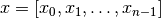as: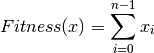Example

>>> import mlrose
>>> import numpy as np
>>> fitness = mlrose.OneMax()
>>> state = np.array([0, 1, 0, 1, 1, 1, 1])
>>> fitness.evaluate(state)
5


Note

The One Max fitness function is suitable for use in either discrete or continuous-state optimization problems.

evaluate(state)[source]

Evaluate the fitness of a state vector.

Parameters: state (array) – State array for evaluation. fitness (float) – Value of fitness function.
class FlipFlop[source]

Fitness function for Flip Flop optimization problem. Evaluates the fitness of a state vector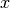as the total number of pairs of consecutive elements of, (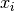and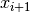) where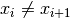.

Example

>>> import mlrose
>>> import numpy as np
>>> fitness = mlrose.FlipFlop()
>>> state = np.array([0, 1, 0, 1, 1, 1, 1])
>>> fitness.evaluate(state)
3


Note

The Flip Flop fitness function is suitable for use in discrete-state optimization problems only.

evaluate(state)[source]

Evaluate the fitness of a state vector.

Parameters: state (array) – State array for evaluation. fitness (float) – Value of fitness function.
class FourPeaks(t_pct=0.1)[source]

Fitness function for Four Peaks optimization problem. Evaluates the fitness of an n-dimensional state vector, given parameter T, as: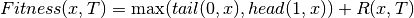where:

•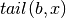is the number of trailing b’s in;
•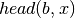is the number of leading b’s in;
•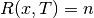, if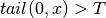and; and
•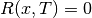, otherwise.
Parameters: t_pct (float, default: 0.1) – Threshold parameter (T) for Four Peaks fitness function, expressed as a percentage of the state space dimension, n (i.e.).

Example

>>> import mlrose
>>> import numpy as np
>>> fitness = mlrose.FourPeaks(t_pct=0.15)
>>> state = np.array([1, 1, 1, 0, 1, 0, 0, 1, 0, 0, 0, 0])
>>> fitness.evaluate(state)
16


References

De Bonet, J., C. Isbell, and P. Viola (1997). MIMIC: Finding Optima by Estimating Probability Densities. In Advances in Neural Information Processing Systems (NIPS) 9, pp. 424–430.

Note

The Four Peaks fitness function is suitable for use in bit-string (discrete-state with max_val = 2) optimization problems only.

evaluate(state)[source]

Evaluate the fitness of a state vector.

Parameters: state (array) – State array for evaluation. fitness (float.) – Value of fitness function.
class SixPeaks(t_pct=0.1)[source]

Fitness function for Six Peaks optimization problem. Evaluates the fitness of an n-dimensional state vector, given parameter T, as:where:

•is the number of trailing b’s in;
•is the number of leading b’s in;
•, if (and) or (and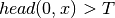); and
•, otherwise.
Parameters: t_pct (float, default: 0.1) – Threshold parameter (T) for Six Peaks fitness function, expressed as a percentage of the state space dimension, n (i.e.).

Example

>>> import mlrose
>>> import numpy as np
>>> fitness = mlrose.SixPeaks(t_pct=0.15)
>>> state = np.array([0, 0, 0, 1, 0, 1, 1, 0, 1, 1, 1, 1])
>>> fitness.evaluate(state)
12


References

De Bonet, J., C. Isbell, and P. Viola (1997). MIMIC: Finding Optima by Estimating Probability Densities. In Advances in Neural Information Processing Systems (NIPS) 9, pp. 424–430.

Note

The Six Peaks fitness function is suitable for use in bit-string (discrete-state with max_val = 2) optimization problems only.

evaluate(state)[source]

Evaluate the fitness of a state vector.

Parameters: state (array) – State array for evaluation. fitness (float) – Value of fitness function.
class ContinuousPeaks(t_pct=0.1)[source]

Fitness function for Continuous Peaks optimization problem. Evaluates the fitness of an n-dimensional state vector, given parameter T, as: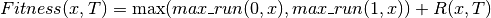where:

•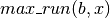is the length of the maximum run of b’s in;
•, if (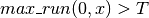and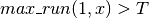); and
•, otherwise.
Parameters: t_pct (float, default: 0.1) – Threshold parameter (T) for Continuous Peaks fitness function, expressed as a percentage of the state space dimension, n (i.e.).

Example

>>> import mlrose
>>> import numpy as np
>>> fitness = mlrose.ContinuousPeaks(t_pct=0.15)
>>> state = np.array([0, 0, 0, 1, 0, 0, 0, 0, 0, 1, 1, 1])
>>> fitness.evaluate(state)
17


Note

The Continuous Peaks fitness function is suitable for use in bit-string (discrete-state with max_val = 2) optimization problems only.

evaluate(state)[source]

Evaluate the fitness of a state vector.

Parameters: state (array) – State array for evaluation. fitness (float) – Value of fitness function.
class Knapsack(weights, values, max_weight_pct=0.35)[source]

Fitness function for Knapsack optimization problem. Given a set of n items, where item i has known weight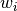and known value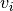; and maximum knapsack capacity,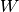, the Knapsack fitness function evaluates the fitness of a state vectoras: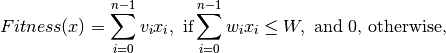wheredenotes the number of copies of item i included in the knapsack.

Parameters: weights (list) – List of weights for each of the n items. values (list) – List of values for each of the n items. max_weight_pct (float, default: 0.35) – Parameter used to set maximum capacity of knapsack (W) as a percentage of the total of the weights list (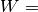max_weight_pcttotal_weight).

Example

>>> import mlrose
>>> import numpy as np
>>> weights = [10, 5, 2, 8, 15]
>>> values = [1, 2, 3, 4, 5]
>>> max_weight_pct = 0.6
>>> fitness = mlrose.Knapsack(weights, values, max_weight_pct)
>>> state = np.array([1, 0, 2, 1, 0])
>>> fitness.evaluate(state)
11


Note

The Knapsack fitness function is suitable for use in discrete-state optimization problems only.

evaluate(state)[source]

Evaluate the fitness of a state vector.

Parameters: state (array) – State array for evaluation. Must be the same length as the weights and values arrays. fitness (float) – Value of fitness function.
class TravellingSales(coords=None, distances=None)[source]

Fitness function for Travelling Salesman optimization problem. Evaluates the fitness of a tour of n nodes, represented by state vector, giving the order in which the nodes are visited, as the total distance travelled on the tour (including the distance travelled between the final node in the state vector and the first node in the state vector during the return leg of the tour). Each node must be visited exactly once for a tour to be considered valid.

Parameters: coords (list of pairs, default: None) – Ordered list of the (x, y) coordinates of all nodes (where element i gives the coordinates of node i). This assumes that travel between all pairs of nodes is possible. If this is not the case, then use distances instead. distances (list of triples, default: None) – List giving the distances, d, between all pairs of nodes, u and v, for which travel is possible, with each list item in the form (u, v, d). Order of the nodes does not matter, so (u, v, d) and (v, u, d) are considered to be the same. If a pair is missing from the list, it is assumed that travel between the two nodes is not possible. This argument is ignored if coords is not None.

Examples

>>> import mlrose
>>> import numpy as np
>>> coords = [(0, 0), (3, 0), (3, 2), (2, 4), (1, 3)]
>>> dists = [(0, 1, 3), (0, 2, 5), (0, 3, 1), (0, 4, 7), (1, 3, 6),
(4, 1, 9), (2, 3, 8), (2, 4, 2), (3, 2, 8), (3, 4, 4)]
>>> fitness_coords = mlrose.TravellingSales(coords=coords)
>>> state = np.array([0, 1, 4, 3, 2])
>>> fitness_coords.evaluate(state)
13.86138...
>>> fitness_dists = mlrose.TravellingSales(distances=dists)
>>> fitness_dists.evaluate(state)
29


Note

1. The TravellingSales fitness function is suitable for use in travelling salesperson (tsp) optimization problems only.
2. It is necessary to specify at least one of coords and distances in initializing a TravellingSales fitness function object.
evaluate(state)[source]

Evaluate the fitness of a state vector.

Parameters: state (array) – State array for evaluation. Each integer between 0 and (len(state) - 1), inclusive must appear exactly once in the array. fitness (float) – Value of fitness function. Returns np.inf if travel between two consecutive nodes on the tour is not possible.
class Queens[source]

Fitness function for N-Queens optimization problem. Evaluates the fitness of an n-dimensional state vector, whererepresents the row position (between 0 and n-1, inclusive) of the ‘queen’ in column i, as the number of pairs of attacking queens.

Example

>>> import mlrose
>>> import numpy as np
>>> fitness = mlrose.Queens()
>>> state = np.array([1, 4, 1, 3, 5, 5, 2, 7])
>>> fitness.evaluate(state)
6


References

Russell, S. and P. Norvig (2010). Artificial Intelligence: A Modern Approach, 3rd edition. Prentice Hall, New Jersey, USA.

Note

The Queens fitness function is suitable for use in discrete-state optimization problems only.

evaluate(state)[source]

Evaluate the fitness of a state vector.

Parameters: state (array) – State array for evaluation. fitness (float) – Value of fitness function.
class MaxKColor(edges)[source]

Fitness function for Max-k color optimization problem. Evaluates the fitness of an n-dimensional state vector, whererepresents the color of node i, as the number of pairs of adjacent nodes of the same color.

Parameters: edges (list of pairs) – List of all pairs of connected nodes. Order does not matter, so (a, b) and (b, a) are considered to be the same.

Example

>>> import mlrose
>>> import numpy as np
>>> edges = [(0, 1), (0, 2), (0, 4), (1, 3), (2, 0), (2, 3), (3, 4)]
>>> fitness = mlrose.MaxKColor(edges)
>>> state = np.array([0, 1, 0, 1, 1])
>>> fitness.evaluate(state)
3


Note

The MaxKColor fitness function is suitable for use in discrete-state optimization problems only.

evaluate(state)[source]

Evaluate the fitness of a state vector.

Parameters: state (array) – State array for evaluation. fitness (float) – Value of fitness function.
class CustomFitness(fitness_fn, problem_type='either', **kwargs)[source]

Class for generating your own fitness function.

Parameters: fitness_fn (callable) – Function for calculating fitness of a state with the signature fitness_fn(state, **kwargs). problem_type (string, default: ‘either’) – Specifies problem type as ‘discrete’, ‘continuous’, ‘tsp’ or ‘either’ (denoting either discrete or continuous). kwargs (additional arguments) – Additional parameters to be passed to the fitness function.

Example

>>> import mlrose
>>> import numpy as np
>>> def cust_fn(state, c): return c*np.sum(state)
>>> kwargs = {'c': 10}
>>> fitness = mlrose.CustomFitness(cust_fn, **kwargs)
>>> state = np.array([1, 2, 3, 4, 5])
>>> fitness.evaluate(state)
150

evaluate(state)[source]

Evaluate the fitness of a state vector.

Parameters: state (array) – State array for evaluation. fitness (float) – Value of fitness function.